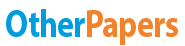# Determining the Hardness of Water Lab Report

Essay by   •  September 18, 2017  •  Lab Report  •  1,397 Words (6 Pages)  •  699 Views

## Essay Preview: Determining the Hardness of Water Lab Report

Report this essay
Page 1 of 6

Determining the Hardness of Water Lab Report

Abstract

The water hardness of a prepared unknown water sample was determined by comparing test results of titration of a prepared 500 mL of 0.004 M disodium EDTA solution. The tests conducted used Eriochrome Black T as the color indicator for the titration tests using the prepared Na2EDTA. It was determined that the prepared unknown sample of water had a water hardness of 252.4 ppm.

Introduction

Determining the hardness of water in a certain region is important. The southwestern part of the U.S has some of the hardest water in the U.S. Arizona for example has moderately hard (3.5-7 grains per gallon) – extremely hard (10.5+ grains per gallon) water depending on where the sample is being taken from within the state. On average, hardness in Arizona water is about 300 ppm (Arizona Republic 83). Usually water hardness is reported as mg CaCO3/L of solution, it can also be reported as parts per million, which is how it will be reported in this lab. Studies have shown that water hardness has no real side effects to health, but the problem presents itself as leaving a residue buildup wherever it goes (faucets, fixtures, coffee makers, pools), as well as poor soap performance. A big problem in Arizona is hard water in pools, According to The Arizona Republic, “The No. 1 problem in many pools is hard water resulting in an oversaturated mineral content” (Arizona Republic 360).

In this lab a disodium EDTA (Na2EDTA) solution will be used to determine the metal ion impurities in water. This will be done through a complexometric titration. A complexometric titration means that a formation of color will be used to determine the end point of the titration. The indicator being used is Eriochrome Black T. Ammonia/ammonium will be added as a buffer to confirm the calcium ions stay in the solution and that the eriochrome black T works properly.

The molarity of Na2EDTA can be found using this equation:

 10 mL CaCO3 soln[pic 1] 1.00 g CaCO3[pic 2] 1 mol CaCO3[pic 3] 1 mol Na2EDTA X mL Na2EDTA soln 1.00 L CaCO3 soln 100.1 g CaCO3 1 mol CaCO3

The hardness of water can be found using this equation:

 X mL Na2EDTA soln X mol Na2EDTA 1 mol CaCO3 100.1 g CaCO3 0.02500 L CaCO3 soln[pic 4] 1 L Na2EDTA[pic 5] 1 mol Na2EDTA[pic 6] 1 mol CaCO3

Water hardness will be presented in ppm=       mg CaCO3[pic 7]

1 L CaCO3 solution

Absolute deviation can be found with this equation:[Na2EDTA mean]-[Na2EDTA n]

Where n = molarity of trial

Estimated precision (ppt) can be calculated with this equation:

Σ absolute deviations/3        [pic 8]

Na2EDTA mean

Procedure

Prepare 500 mL of 0.004 M disodium EDTA solution. Standardize the Na2EDTA solution using a stock calcium ion solution (1.000 g CaCO3/L solution) as the primary standard. Read and record volume delivered, repeat two more times. Calculate the molarity of your disodium EDTA from each titration, average the molarities from each titration and calculate your precision. Choose a prepared unknown water sample as provided. Record the unknown number, then titrate this water sample with your standardized disodium EDTA solution. Record volume delivered and repeat titration two more times. Calculate the hardness (mg CaCO3/L) of the prepared water sample from each titration. Calculate the average water hardness and the experimental precision from the three trials. Compare the results to the expected range for municipal water hardness.

Results and Discussion

The unknown water sample used in this experiment was #62. To determine the water hardness of the sample, a known Na2EDTA solution had to be created first. After adding 0.7754 g Na2EDTA to 500 mL of Deionized water, the solution was then titrated with a 1.000 g CaCo3/L solution to find the Molar concentration of the Na2EDTA solution. After recording the volume of Na2EDTA solution delivered (figure 1) this equation was used to find the Molar concentration of Na2EDTA solution.

 0.01 L CaCO3[pic 9][pic 10] 1.0 g CaCO3[pic 11][pic 12] 1 mol CaCO3[pic 13][pic 14] 1 mol Na2EDTA 0.0219 L Na2EDTA[pic 15] 1.0 L CaCO3[pic 16][pic 17] 100.1 g CaCO3[pic 18][pic 19] 1 mol CaCO3[pic 20]

= 0.004562 M Na2EDTA

After finding the molarity of the Na2EDTA solution (figure 1), absolute deviations (Figure 1) and the estimated precision was calculated. The absolute deviations were found by subtracting each trials found Na2EDTA molarity and subtracting it from the average molarity of all 3 trials, then taking the absolute value to find the answer. The estimated precision was calculated with this equation.

...

...